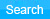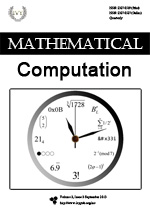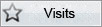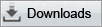# HomePage >> Journals >> Mathematical Computation## Mathematical Computation

Mathematical Computation (Yearly) is an international comprehensive professional academic journal of Ivy Publisher, concerning the development of mathematical theory and computing application on the combination of mathematical theory and modern industrial technology. The main focus of the journal is the academic papers and comments of latest theoretical and apolitical mathematics improvement in the fields of nature science, engineering technology... [More] Mathematical Computation (Yearly) is an international comprehensive professional academic journal of Ivy Publisher, concerning the development of mathematical theory and computing application on the combination of mathematical theory and modern industrial technology. The main focus of the journal is the academic papers and comments of latest theoretical and apolitical mathematics improvement in the fields of nature science, engineering technology, economy and science, report of latest research result, aiming at providing a good communication platform to transfer, share and discuss the theoretical and technical development of mathematics theory development for professionals, scholars and researchers in this field, reflecting the academic front level, promote academic change and foster the rapid expansion of mathematics theory and application technology.

The journal receives manuscripts written in Chinese or English. As for Chinese papers, the following items in English are indispensible parts of the paper: paper title, author(s), author(s)'affiliation(s), abstract and keywords. If this is the first time you contribute an article to the journal, please format your manuscript as per the sample paper and then submit it into the online submission system. Accepted papers will immediately appear online followed by printed hard copies by Ivy Publisher globally. Therefore, the contributions should not be related to secret. The author takes sole responsibility for his views.

ISSN Print:2327-0519

ISSN Online:2327-0527

Email:mc@ivypub.org

Website: http://www.ivypub.org/mc/00

## Paper Infomation

Three Modified Efficient Iterative Methods for Non-linear Equations

Full Text(PDF, 551KB)

Author: Liang Fang, Lili Ni, Rui Chen

Keywords: Iterative Method，Non-linear Equation，Order of Convergence，Newton's Method，Efficiency Index

 A.M. Ostrowski, Solution of Equations in Euclidean and Banach Space, Academic Press, New York, 1973.

 A.N. Muhammad and A. Faizan: Fourth-order convergent iterative method for nonlinear equation, Appl. Math. Comput. 182 (2006), p. 1149-1153.

 A.N. Muhammad and I.N. Khalida: Modified iterative methods with cubic convergence for solving nonlinear equations, Appl. Math. Comput. 184 (2007), p. 322-325.

 C. Chun: Some second-derivative-free variants of super-Halley method with fourth-order convergence,Appl. Math. Comput. 192 (2007), p. 537-541.

 C. Chun: A simply constructed third-order modifications of Newton's method, J. Comput. Appl. Math. 219(2008), p. 81-89.

 F.A. Potra, Potra-Pták: Nondiscrete induction and iterative processes, Research Notes in Mathematics, Vol. 103, Pitman, Boston, 1984.

 J.F. Traub: Iterative Methods for Solution of Equations, Prentice-Hall, Englewood Clis, NJ(1964)

 J. Kou: The improvements of modified Newton's method, Appl. Math. Comput. 189 (2007), p. 602-609.

 J. Kou, Y. Li, The improvements of Chebyshev-Halley methods with fifth-order convergence, Appl. Math. Comput., 188 (2007), 143-147.

 L. Fang and G. He: Some modifications of Newton's method with higher-order convergence for solving nonlinear equations, J. Comput. Appl. Math. 228 (2009), p. 296-303.

 L. Fang, G. He and Z. Hu: A cubically convergent Newton-type method under weak conditions, J. Comput. Appl. Math. 220 (2008), p. 409-412.

 L. Fang, L. Sun and G. He: An efficient Newton-type method with fifth-order for solving nonlinear equations, Comput. Appl. Math. 27 (2008), p. 269-274.

 H.H.H. Homeier, A modified Newton method for rootfinding with cubic convergence, J. Comput. Appl. Math., 157 (2003), 227-230.

 J. Kou, Y. Li, X. Wang, Some modifications of Newton’s method with fifth-order convergence, Appl. Math. Comput., 209(2007), 146-152.

 J.R. Sharma, R.K. Guha, A family of modified Ostrowski methods with accelerated sixth-order convergence, Appl. Math.Comput., 190(2007), 111-115.

 M.A. Noor and K.I. Noor: Fifth-order iterative methods for solving nonlinear equations, Appl. Math. Comput. 188 (2007), p. 406-410

 M. Grau and J.L. Diaz-Barrero:An improvement to Ostrowski root-finding method, Appl. Math. Comput. 173 (2006), p. 450-456.

 M. Grau, M. Noguera, A Variant of Cauchy's Method with Accelerated Fifth-Order Convergence, Applied MathematicsLetters., 17 (2004), 509-517.